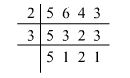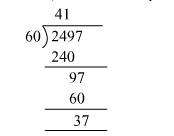# Find the least number which should be added to 2497Question:

Find the least number which should be added to 2497 so that the sum is exactly divisible by 5, 6, 4 and 3.

Solution:

We find the LCM of 5, 6, 4 and 3 first.​So, $\operatorname{LCM}(5,6,4,3)=2 \times 2 \times 3 \times 5=60$

Now, divide 2497 by 60, we getTo make the number completely divisible by 60, we must add a number that would make the remainder equal to 60.
Therefore, the number that must be added is 60 -">- 37 = 23
Hence, 23 must be added to 2497.
So, the number exactly divisible by 5, 6, 4 and 3 is 2497 + 23 = 2520